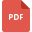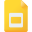# algebra tutorialsHow to Solve Basic Algebraic Equations
Mathematics, Creative Teaching, AlgebraLearn Algebraic Expression Simplification
Expressions, Grade 3, United States, AlgebraCombining and Simplifying Algebraic Expressions
Algebra, Grade 8, United States, MathematicsThe Implementation of Vedic Mathematics to Algebra and Geometry
Vedic Mathematics, Class 10, India, Algebra, GeometrySimplifying Algebraic Expressions
Mathematics, Grade 7, United States, AlgebraComplex Fractions: Objectives and Examples
Fractions and Multiplication, High School, United States, Mathematics, AlgebraSimplification of Algebraic Expressions
Algebra, Grade 8, United States, MathematicsAlgebraic Expressions and Number Sentences
Algebra, Grade 3, United States, Expressions, Number SentencesApplications of Vedic Mathematics in Algebra
Vedic Mathematics, Class 9, India, Basic MathExpanding and simplifying algebraic expressions
Mathematics, Grade 7, United States, AlgebraSubstitution into Algebraic Expressions
Mathematics, Grade 8, United States, AlgebraElementary Algebra on Vedic Mathematics
Vedic Mathematics, Class 9, India, Basic MathIntegration Using Tables and Computer Algebra Systems
Integral Calculus, High School, United States, Computer Algebra27 - Introducing to Algebraic Expressions
Algebra, Grade 8, United States, MathematicsAlgebra worksheet
Mathematics, Grade 7, United States, AlgebraAlgebraic Expressions Practice Worksheet
Mathematics, Grade 8, United States, AlgebraSimplifying Easy Algebraic Fractions
Algebra, Grade 8, United States, MathematicsOperations with Algebraic Expressions
Mathematics, Grade 7, United States, AlgebraAlgebraic expressions
Mathematics, Grade 7, United States, AlgebraExpanding and Removing Brackets
Mathematics, Grade 7, United States, Algebra